# HSSlive: Plus One & Plus Two Notes & Solutions for Kerala State Board

## AP Board Class 7 Maths Chapter 1 Integers Ex 3 Textbook Solutions PDF: Download Andhra Pradesh Board STD 7th Maths Chapter 1 Integers Ex 3 Book AnswersAP Board Class 7 Maths Chapter 1 Integers Ex 3 Textbook Solutions PDF: Download Andhra Pradesh Board STD 7th Maths Chapter 1 Integers Ex 3 Book Answers

## Andhra Pradesh State Board Class 7th Maths Chapter 1 Integers Ex 3 Books Solutions

 Board AP Board Materials Textbook Solutions/Guide Format DOC/PDF Class 7th Subject Maths Chapters Maths Chapter 1 Integers Ex 3 Provider Hsslive

2. Click on the Andhra Pradesh Board Class 7th Maths Chapter 1 Integers Ex 3 Answers.
3. Look for your Andhra Pradesh Board STD 7th Maths Chapter 1 Integers Ex 3 Textbooks PDF.

## AP Board Class 7th Maths Chapter 1 Integers Ex 3 Textbooks Solutions with Answer PDF Download

Find below the list of all AP Board Class 7th Maths Chapter 1 Integers Ex 3 Textbook Solutions for PDF’s for you to download and prepare for the upcoming exams:

Question 1.
Represent the following subtractions on the number line.
(i) 7 – 2
(ii) 8 – ( – 7)
(iii) 3 – 7
(iv) 1 5 – 14
(v) 5 – ( – 8)
(vi) ( – 2) – ( – 1)
Solution:
(i) 7 – 2 = 5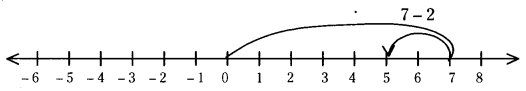(ii) 8 – ( – 7) = 15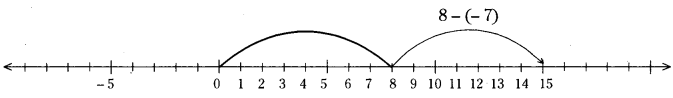(iii) 3 – 7 = -4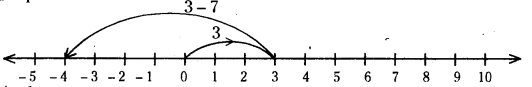(iv) 1 5 – 14 = 1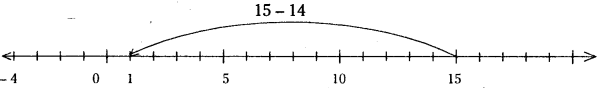(v) 5 – ( – 8) = 13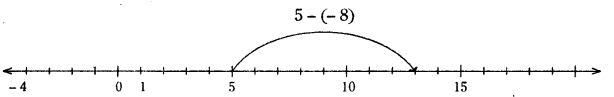(vi) ( – 2) – ( – 1) = -2 + 1 = -1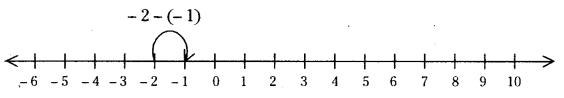Question 2.
Solve the following.
(i) 17 – ( – 14)
(ii) 13 – ( – 8)
(iii) 19 – ( – 5)
(iv) 15 – 28
(v) 25 – 33
(vi) 80 – ( – 50)
(vii) 150 – 75
(viii) 32 – ( – 18)
Solution:
(i) 17 – ( – 14) = 31
(ii) 13 – ( – 8) = 21
(iii) 19 – ( – 5) = 24
(iv) 15 – 28 = -13
(v) 25 – 33 = -8
(vi) 80 – ( – 50) = 130
(vii) 150 – 75 = 75
(viii) 32 – ( – 18) = 50

Question 3.
Express ‘ – 6’ as the difference between a negative integer and a whole number.
Solution:
– 6=( – 4) – (2) ( – 5) – (1)=( – 2) – (4)

## Andhra Pradesh Board Class 7th Maths Chapter 1 Integers Ex 3 Textbooks for Exam Preparations

Andhra Pradesh Board Class 7th Maths Chapter 1 Integers Ex 3 Textbook Solutions can be of great help in your Andhra Pradesh Board Class 7th Maths Chapter 1 Integers Ex 3 exam preparation. The AP Board STD 7th Maths Chapter 1 Integers Ex 3 Textbooks study material, used with the English medium textbooks, can help you complete the entire Class 7th Maths Chapter 1 Integers Ex 3 Books State Board syllabus with maximum efficiency.

## FAQs Regarding Andhra Pradesh Board Class 7th Maths Chapter 1 Integers Ex 3 Textbook Solutions

#### Can we get a Andhra Pradesh State Board Book PDF for all Classes?

Yes you can get Andhra Pradesh Board Text Book PDF for all classes using the links provided in the above article.

## Important Terms

Andhra Pradesh Board Class 7th Maths Chapter 1 Integers Ex 3, AP Board Class 7th Maths Chapter 1 Integers Ex 3 Textbooks, Andhra Pradesh State Board Class 7th Maths Chapter 1 Integers Ex 3, Andhra Pradesh State Board Class 7th Maths Chapter 1 Integers Ex 3 Textbook solutions, AP Board Class 7th Maths Chapter 1 Integers Ex 3 Textbooks Solutions, Andhra Pradesh Board STD 7th Maths Chapter 1 Integers Ex 3, AP Board STD 7th Maths Chapter 1 Integers Ex 3 Textbooks, Andhra Pradesh State Board STD 7th Maths Chapter 1 Integers Ex 3, Andhra Pradesh State Board STD 7th Maths Chapter 1 Integers Ex 3 Textbook solutions, AP Board STD 7th Maths Chapter 1 Integers Ex 3 Textbooks Solutions,
Share: